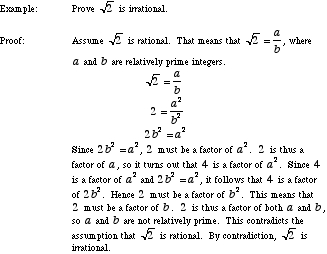index: click on a letter A B C D E F G H I J K L M N O P Q R S T U V W X Y Z A to Z index index: subject areas numbers & symbols sets, logic, proofs geometry algebra trigonometry advanced algebra & pre-calculus calculus advanced topics probability & statistics real world applications multimedia entrieswww.mathwords.com about mathwords website feedback

 Proof by Contradiction Indirect Proof Proving a conjecture by assuming that the conjecture is false. If this assumption leads to a contradiction, the original conjecture must have been true. This technique employs the logical method known as modus tolens.See also